Difference between revisions of "Work"

The mechanical work, W, performed upon a gas is given by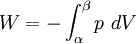$W = - \int_\alpha^\beta p ~dV$

where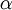$\alpha$ and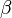$\beta$ are points in state space, p is the pressure and V is the volume. The mechanical work done by the gas has the opposite sign (a common source of mistakes).

For reversible changes,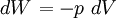$\left.dW\right.=-p~dV$

and for irreversible changes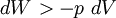$\left.dW\right. > -p~dV$